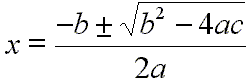﻿ Quadratic Solver | Zona Land Education

0 = ax2 + bx + c

 a = b = c =

The above program will calculate solutions to the quadratic equation.

• Enter values for the coefficients a, b, and c.
• Click the 'Calculate' button.
• The roots of the quadratic equation will pop up.

These values for x are calculated using the solution to the quadratic equation, which is:Of course, the coefficient 'a' must be non-zero for this to be a quadratic equation. Solutions are only calculated when a is non-zero.

Click this button to see the actual JavaScript code that executes on this page:

Custom Search